### IMO Shortlist 1987 problem 8

Kvaliteta:
Avg: 0,0
Težina:
Avg: 0,0
(a) Let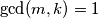$\gcd(m, k) = 1$. Prove that there exist integers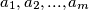$a_1, a_2, . . . , a_m$ and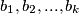$b_1, b_2, . . . , b_k$ such that each product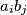$a_ib_j$ (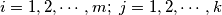$i = 1, 2, \cdots ,m; \ j = 1, 2, \cdots, k$) gives a different residue when divided by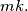$mk.$

(b) Let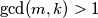$\gcd(m, k) > 1$. Prove that for any integers$a_1, a_2, . . . , a_m$ and$b_1, b_2, . . . , b_k$ there must be two products$a_ib_j$ and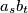$a_sb_t$ (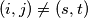$(i, j) \neq (s, t)$) that give the same residue when divided by$mk.$

Proposed by Hungary.
Izvor: Međunarodna matematička olimpijada, shortlist 1987